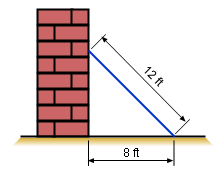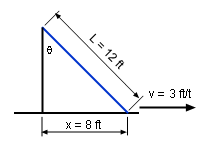Ch 7. Inverse Functions Multimedia Engineering Math InverseFunctions ExponentialFunctions Inverse Trig.Functions
 Chapter 1. Limits 2. Derivatives I 3. Derivatives II 4. Mean Value 5. Curve Sketching 6. Integrals 7. Inverse Functions 8. Integration Tech. 9. Integrate App. 10. Parametric Eqs. 11. Polar Coord. 12. Series Appendix Basic Math Units Search eBooks Dynamics Fluids Math Mechanics Statics Thermodynamics Author(s): Hengzhong Wen Chean Chin Ngo Meirong Huang Kurt Gramoll ©Kurt GramollMATHEMATICS - CASE STUDY IntroductionThe State of the Ladder In order to hang a painting on a well, Jim moved a ladder and leaned it against the wall. However, the ladder started to slide away from the wall when he started clim the ladder. What is known: The length of the ladder is 12 ft. The ladder horizontal speed at the base is 3 ft/s. The distance between the bottom of the ladder and the base of the wall is 8 ft. QuestionsSimplified Ladder State How fast does the angle, θ, between the ladder and the wall change when the bottom of the ladder is 8 ft from the base of the wall? Approach Using the inverse trigonometric function to calculate the rate of change of the angle θ.